Courses

# Test: ILD & Rolling Loads - 3

## 10 Questions MCQ Test Structural Analysis | Test: ILD & Rolling Loads - 3

Description
This mock test of Test: ILD & Rolling Loads - 3 for GATE helps you for every GATE entrance exam. This contains 10 Multiple Choice Questions for GATE Test: ILD & Rolling Loads - 3 (mcq) to study with solutions a complete question bank. The solved questions answers in this Test: ILD & Rolling Loads - 3 quiz give you a good mix of easy questions and tough questions. GATE students definitely take this Test: ILD & Rolling Loads - 3 exercise for a better result in the exam. You can find other Test: ILD & Rolling Loads - 3 extra questions, long questions & short questions for GATE on EduRev as well by searching above.
QUESTION: 1

Solution:
QUESTION: 2

Solution:
QUESTION: 3

### Which one of the following is the influence line for reaction at A of the beam shown in the figure?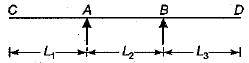Solution:

Using Castigliano’s second theorem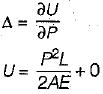There is no force in member (1) - (2),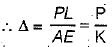QUESTION: 4

Select the correct influence line diagram for shear force at x of the following beam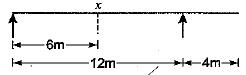Solution:

Maximum shear force is at either of the support due to a point load

QUESTION: 5

The maximum bending moment at the left quarter point of a simple beam due to crossing of UDL of length shorter than the span in the direction left to right, would occur after the load had just crossed the section by

Solution:

The load should be positioned such that section divides the span and load in the same ratio.

QUESTION: 6

For the continuous beam shown in figure, the influence line diagram for support reaction at D is best represented as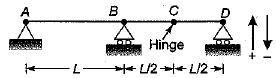Solution:

The ILD for support reaction at D can be obtained by:giving unit displacement in the direction of reaction. The deflected shape of beam will represent ILD as in figure (c).

QUESTION: 7

Consider the following statements:
1. Influence Line Diagram (ILD) for SF at the fixed end of a cantilever and SFD due to unit load at the free end are same.
2. ILD for BM at the fixed end of a cantilever and BMD due to unit load at the free end are same.
Which of these statements is/are correct?

Solution:

ILD for BM at fixed end will have maximum ordinate when the unit load is at free end. While the BMD due to unit load at free end will have zero ordinate at free end and maximum ordinate at fixed end.

QUESTION: 8

The influence line diagram (ILD) shown is for the member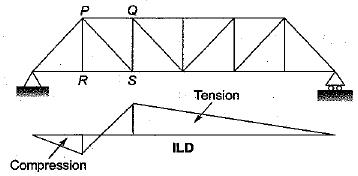Solution:
QUESTION: 9

A uniformly distributed line load of 60 kN per metre run of length 5 meters on a girder of span 16 metres. What is the maximum positive shear force at a section 6 metres from the left end.

Solution:

We must first draw the influence line diagram for the SF at the section D,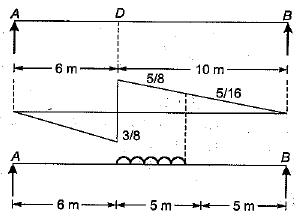For maximum positive SF at D, the loading should be applied as shown in the figure.
Maximum positive = load x area of ILD SF at D intensity covered by the load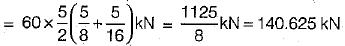QUESTION: 10

The wheel loads 200 kN and 80 kN spaced 2 m apart move on the span of girder of span 16 metres. What is the maximum bending moment that will occur at a section 6 metres from the left end.

Solution: